# ILTS Mathematics: Linear Relations & Functions Chapter Exam

Exam Instructions:

Choose your answers to the questions and click 'Next' to see the next set of questions. You can skip questions if you would like and come back to them later with the yellow "Go To First Skipped Question" button. When you have completed the practice exam, a green submit button will appear. Click it to see your results. Good luck!

### Page 1

#### Question 5 5. Linearize f(x) about the point x=0: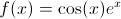### Page 2

#### Question 6 6. Which equation(s) need to be rewritten in this linear system before we can write it in augmented matrix form?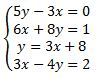### Page 3

#### Question 14 14. Linearize f(x) about the point x=0: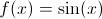### Page 4

#### Question 18 18. Linearize f(x) about the point x: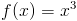#### Question 19 19. Linearize f(x) about the point x=pi/2.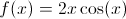### Page 5

#### Question 22 22. Linearize f(x) about the point x: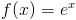#### Question 23 23. Which system of equations is represented by the following matrix? (Think of the vertical line as the equal sign.)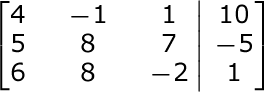#### Question 24 24. Which of the following is an equation included in this matrix?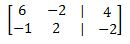### Page 6

#### Question 26 26. What is the complete list of equations included in this augmented matrix?#### Question 28 28. Rewrite the 3rd equation in proper form so that we can turn it into augmented matrix form.#### ILTS Mathematics: Linear Relations & Functions Chapter Exam Instructions

Choose your answers to the questions and click 'Next' to see the next set of questions. You can skip questions if you would like and come back to them later with the yellow "Go To First Skipped Question" button. When you have completed the practice exam, a green submit button will appear. Click it to see your results. Good luck!

Support